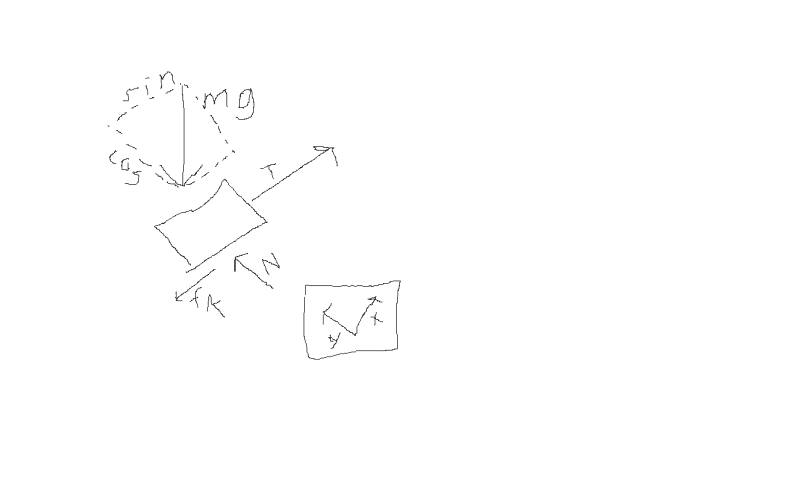# Vector components for custom coordinate axes

• alsvt

#### alsvt

I'm having trouble finding information online regarding figuring out vector components when you set up your own axes. All examples just show a basic x-y coordinate system where the cos and sin components of the vector are obvious.

However, suppose you have a problem where, for example you have something like this (in free body diagram form)...I set up a little FBD representing a box being pulled up an incline. You have the normal force, the tension force, and friction all matching up with the coordinate axis I put in the box below. However, weight (mg) doesn't match up so when you find the sum of the forces you have to use component vectors for it.

I've identified the correct components, but I'm not sure why they are that. As you can see, cos would be used for gravity in the y direction and sin would be used for gravity in the x direction. Can someone explain how to figure out components when it isn't as simple as a vector pointing straight out from an origin? I think the problem I'm having is identifying the location of the angle.

P.S. I wasn't sure whether this is considered "homework" because it isn't a homework problem. It is a conceptual question.

Last edited:
Can someone explain how to figure out components when it isn't as simple as a vector pointing straight out from an origin? I think the problem I'm having is identifying the location of the angle.
Hi alsvt. This is the correct place to post this type of question.

Perhaps you don't realize that for purposes of resolving a vector into its components, you can always draw a second pair of x and y axes parallel to the original axes and such that they intersect at the tail of the vector. In other words, you can translate the coordinate axes parallel to themselves so that the origin coincides with the tail of the vector in question. Then you can use trigonometry to find the components as you have correctly done here.